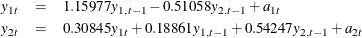The VARMAX Procedure

Dynamic Simultaneous Equations Modeling

In the econometrics literature, the VARMAX(p,q,s) model is sometimes written in a form that is slightly different than the one shown in the previous section. This alternative form is referred to as a dynamic simultaneous equations model or a dynamic structural equations model.

Since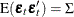is assumed to be positive-definite, there exists a lower triangular matrixwith ones on the diagonals such that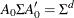, where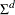is a diagonal matrix with positive diagonal elements.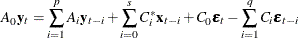where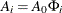,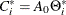,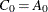, and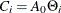.

As an alternative form,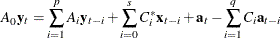where,,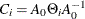, and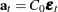has a diagonal covariance matrix. The PRINT=(DYNAMIC) option returns the parameter estimates that result from estimating the model in this form.

A dynamic simultaneous equations model involves a leading (lower triangular) coefficient matrix for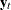at lag 0 or a leading coefficient matrix for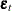at lag 0. Such a representation of the VARMAX(p,q,s) model can be more useful in certain circumstances than the standard representation. From the linear combination of the dependent variables obtained by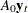, you can easily see the relationship between the dependent variables in the current time.

The following statements provide the dynamic simultaneous equations of the VAR(1) model.

proc iml;
sig = {1.0  0.5, 0.5 1.25};
phi = {1.2 -0.5, 0.6 0.3};
/* simulate the vector time series */
call varmasim(y,phi) sigma = sig n = 100 seed = 34657;
cn = {'y1' 'y2'};
create simul1 from y[colname=cn];
append from y;
quit;

data simul1;
set simul1;
date = intnx( 'year', '01jan1900'd, _n_-1 );
format date year4.;
run;

proc varmax data=simul1;
model y1 y2 / p=1 noint print=(dynamic);
run;


This is the same data set and model used in the section Getting Started: VARMAX Procedure. You can compare the results of the VARMA model form and the dynamic simultaneous equations model form.

Figure 35.25: Dynamic Simultaneous Equations (DYNAMIC Option)

The VARMAX Procedure

Covariances of Innovations
Variable y1 y2
y1 1.28875 0.00000
y2 0.00000 1.29578

AR
Lag Variable y1 y2
0 y1 1.00000 0.00000
y2 -0.30845 1.00000
1 y1 1.15977 -0.51058
y2 0.18861 0.54247

Dynamic Model Parameter Estimates
Equation Parameter Estimate Standard
Error
t Value Pr > |t| Variable
y1 AR1_1_1 1.15977 0.05508 21.06 0.0001 y1(t-1)
AR1_1_2 -0.51058 0.07140 -7.15 0.0001 y2(t-1)
y2 AR0_2_1 0.30845       y1(t)
AR1_2_1 0.18861 0.05779 3.26 0.0015 y1(t-1)
AR1_2_2 0.54247 0.07491 7.24 0.0001 y2(t-1)

In Figure 35.4 in the section Getting Started: VARMAX Procedure, the covariance ofestimated from the VARMAX model form is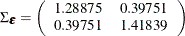Figure 35.25 shows the results from estimating the model as a dynamic simultaneous equations model. By the decomposition of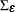, you get a diagonal matrix (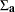) and a lower triangular matrix () such as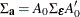where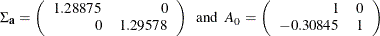The lower triangular matrix () is shown in the left side of the simultaneous equations model. The parameter estimates in equations system are shown in the right side of the two-equations system.

The simultaneous equations model is written as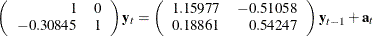The resulting two-equation system can be written as